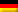Boiling temperatures of the pure substances:

ϑB = 80,1 °C and ϑT = 111 °C (p. 110/111)

Boiling temperatures in Kelvin:

TB = 80,1 + 273,15 = 353,25 K
TT = 111 + 273,15 = 384,15 K

Rose separation factor (p. 256):

Function equation for the equilibrium curve (p. 256):

For example for the substance quantity proportion in the feed:

Minimum return ratio (p. 257):

Selected (p. 256): v = 2 · vmin = 2 · 2,39 = 4,78

Function equation for the gain line (p. 256):

or straight line between the ordinate intersection and the intersection of the perpendicular over xD with the diagonal.

For the ordinate intersection (p. 256):

Point of intersection with the diagonal: yD = xD

Intersection straight line is, since inlet boils liquid, the vertical above xZ . The output straight line is constructed between the intersection point of the intersection point straight line with the reinforcement straight line and the intersection point of the perpendicular above xB with the diagonal.

Drawing the steps between equilibrium curve and gain line and equilibrium curve and output line, starting from xD to over xB . There are 7 theoretical stages in the amplifier section and 4stages in the output section (without consideration of efficiency and buffer floors).

Dieser Beitrag ist auch verfügbar auf:Deutsch (German)